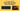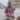# array.sort() Does Not Simply Sort Numbers in JavaScript

Posted January 26, 2021Start discussion

In JavaScript, the `array.sort()` method sorts the array. Let’s use it to sort some numbers:

``````const numbers = [10, 5, 11];

numbers.sort(); // => [10, 11, 5]``````

Hm… `numbers.sort()` returns `[10, 11, 5]` — which doesn’t look like a sorted array in ascrending order.

Why does `array.sort()` method, when invoked without arguments, doesn’t sort the numbers as expected? Let’s find the answer.

## 1. array.sort() without arguments

`array.sort()` is a method on array instance that sorts the array in place (mutates the original array) and returns the sorted array.

When called without arguments, the array items are transformed to strings and sorted… alphabetically.

For example, let’s sort an array of names:

``````const names = ['joker', 'batman', 'catwoman'];

names.sort(); // => ['batman', 'catwoman', 'joker']``````

The names are sorted alphabetically: `['batman', 'catwoman', 'joker']`.

Unfortunately, invoking the method on numbers performs the same alphabetical sorting:

``````const numbers = [10, 5, 11];

numbers.sort(); // => [10, 11, 5]``````

The method returns the array `[10, 11, 5]` having numbers sorted alphabetically, rather than by their numeric value.

## 2. array.sort() with a comparator

Fortunately, `array.sort()` method accepts an optional argument: the comparator function.

``const mutatedArray = array.sort([comparator]);``

Using this function you can control how element are ordered in the array during sorting.

If `comparator(a, b)` returns:

• A negative number `< 0`: then `a` is placed before `b`
• A positive number `> 0`: then `b` is placed before `a`
• Zero `0`: then the position of the compared elements doesn’t change

To correctly sort numbers in ascending order, let’s use the following comparator function:

``````const numbers = [10, 5, 11];

numbers.sort((a, b) => {
if (a < b) {
return -1;
}
if (a > b) {
return 1;
}
return 0;
}); // => [5, 10, 11]``````

`numbers.sort(comparator)` now correctly sorts the numbers: `[5, 10, 11]`.

In a sorted in ascrending order array the smaller number is positioned before a bigger one. That’s the property you need to maintain when coding the comparator function:

• If `a < b` — the function returns `-1`, placing `a` before `b` (e.g. `5 < 8`, thus `5` is before `8`)
• If `a > b` — the function returns `1`, placing `b` before `a` (e.g. `10 > 3`, thus `3` is before `10`)
• If `a === b` — order is not changed.

The comparator function in the previous example is relatively long. Fortunately, it can be simplified by just diffing the arguments:

``````const numbers = [10, 5, 11];

numbers.sort((a, b) => a - b); // => [5, 10, 11]``````

`(a, b) => a - b` is a short comparator to sort numbers. I recommend this form to sort the array of numbers in JavaScript.

## 3. Sorting using a typed array

The typed arrays in JavaScript contain elements of a specific type, e.g. `UInt8`: 8 bit unsigned integers, `Float64`: 64 bit floating point numbers. That’s in contrast to the regular array, where elements can be of any type, or even mix of types.

The good thing about typed arrays is that their `sort()` method by default performs an ordering on the numbers in ascending order.

A contrived approach to sort numbers, without using a comparator function, is to make use of a typed array:

``````const numbers = [10, 5, 11];

const sortedNumbers = [...new Float64Array(numbers).sort()];

sortedNumbers; // => [5, 10, 11]``````

`new Float64Array(numbers)` creates an instance of a typed array initiazed with numbers from `numbers` array.

`new Float64Array(numbers).sort()` sorts in ascending order the numbers of the typed array. Note that a comparator function isn’t needed.

Finally, the spread operator `[...new Float64Array(numbers).sort()]` extracts the sorted numbers from the typed array into a regular array.

## 4. Summary

`array.sort()` method invoked without arguments sorts the elements alphabetically. That’s why using `array.sort()` to sort numbers in ascending order doesn’t work.

But you can indicate a comparator function `array.sort(comparator)` to customize how the elements are sorted. I recommend `numbers.sort((a, b) => a - b)` as one of the shortest way to sort an array of numbers.

Quiz: how would you sort numbers in a descending order? Write your answer in a comment below!

## Quality posts into your inbox

I regularly publish posts containing:

• Important JavaScript concepts explained in simple words
• Overview of new JavaScript features
• How to use TypeScript and typing
• Software design and good coding practices